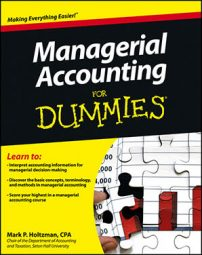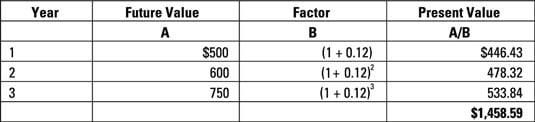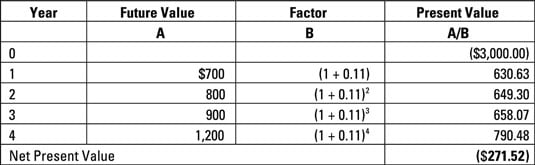##### Managerial Accounting For DummiesMost capital projects are expected to provide a series of cash flows over a period of time. Following are the individual steps necessary for calculating NPV when you have a series of future cash flows: estimating future net cash flows, setting the interest rate for your NPV calculations, computing the NPV of these cash flows, and evaluating the NPV of a capital project.

## How to estimate annual net cash flows

To estimate each year’s net cash flow, add cash inflows from potential revenues to expected savings in materials, labor, and overhead from the new project. Here, include cash savings resulting from incremental costs eliminated by the project. From this sum, subtract any additional costs you’ll need to pay because of the new project. Cash inflows should be set as positive amounts, while cash outflows should be set as negative.

Net means that you’re offsetting each year’s expected cash inflows against its expected cash outflows. If a year’s expected cash inflows exceed the outflows, congratulations! You’re going to have a net cash inflow. On the other hand, if a year’s expected cash inflows fall short of expected outflows, you have an expected net cash outflow.

When estimating annual net cash flows, companies usually account for a depreciation tax shield, which results from tax savings on the depreciation of project assets. To compute this figure, multiply the tax depreciation expense for the year by the company’s expected tax rate that year. Then, because this amount represents tax savings each year, add the result to your expected cash inflows.

## Set the interest rate

Before you can determine the NPV of the cash flows, you need to set an interest rate. For these purposes, companies usually estimate their cost of capital, the average interest rate the company must pay to borrow money from creditors and raise equity from stockholders.

Managers use many different terms to describe the interest rate in a net present value calculation, including the following:

• Cost of capital

• Cutoff rate

• Discount rate

• Hurdle rate

• Required rate of return

## Compute the net present value of a series of annual net cash flows

To determine the present value of these cash flows, use time value of money computations with the established interest rate to convert each year’s net cash flow from its future value back to its present value. Then add these present values together.

Remember to preserve the sign of each year’s net cash flow, such that positive net cash inflows get converted into positive net present values and net cash outflows get converted into negative net present values.

Suppose that Sombrero Corporation expects a new project to yield \$500 one year from now, \$600 in two years, and then \$750 in three years. The company’s cost of capital is 12 percent. The figure illustrates how to convert each of these future values to present value so you can determine total net present value. According to this figure, the total present value of these future cash flows equals \$1,458.59.## How to evaluate the NPV of a capital project

To evaluate the NPV of a capital project, simply estimate the expected net present value of the future cash flows from the project, including the project’s initial investment as a negative amount (representing a payment that needs to be made right now). If a project’s NPV is zero or a positive value, you should accept the project. If the NPV is negative, it represents a loss, and you should reject the project.

Suppose Corporation X is evaluating a project costing \$3,000. Managers expect the project to yield \$700 one year from now, \$800 in two years, \$900 in three years, and \$1,200 in four years. The company’s cost of capital is 11 percent.The net present value of X’s project comes to –\$271.52, indicating that the company would lose \$271.52 on this project. Therefore, managers should reject the project.